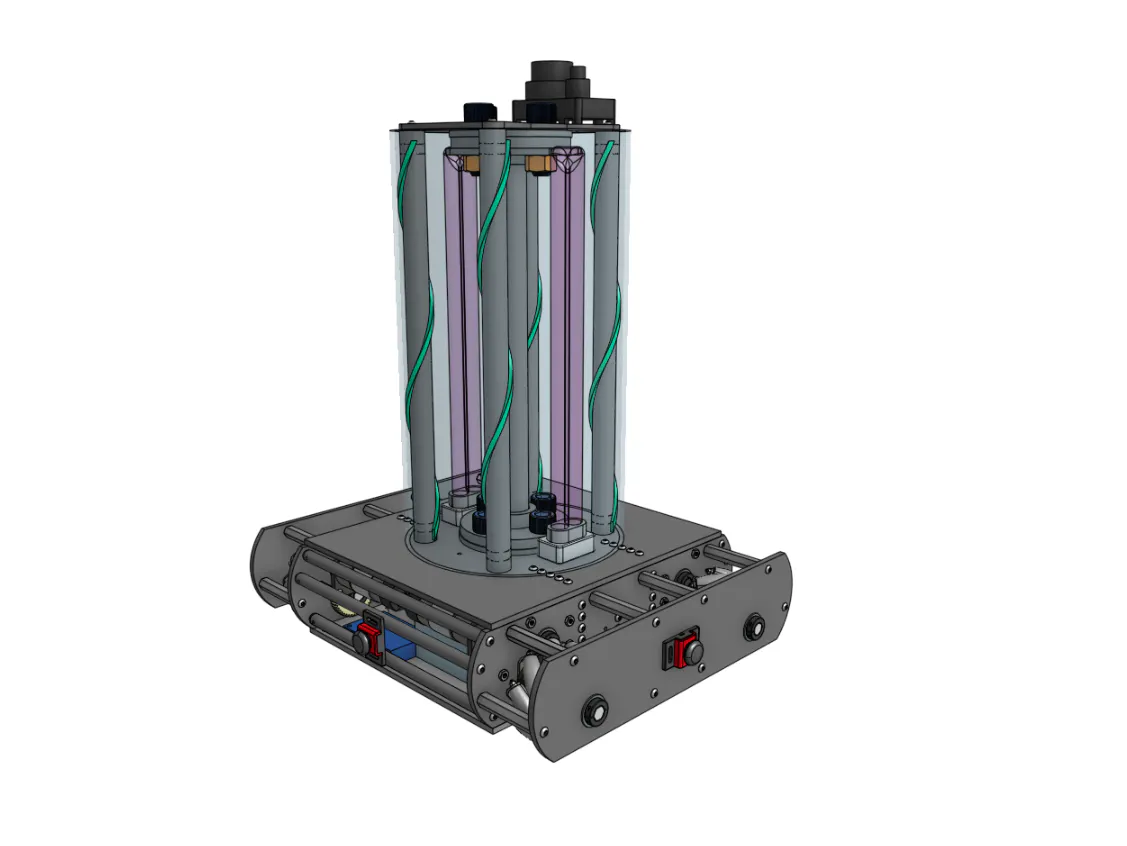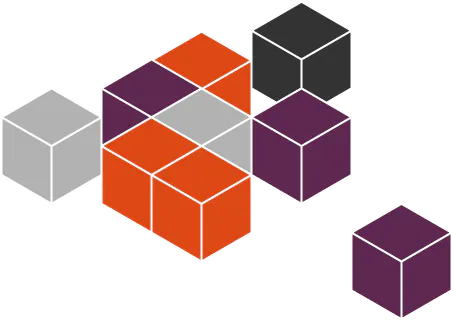Wells: A UV-C Disinfection Robot for Indoor Spaces

Wells is a low-cost UV-C disinfection robot designed to address the need for rapid sanitation of closed spaces due to the COVID-19 Virus

IntermediateShowcase (no instructions)348Things used in this project

×1

Software apps and online servicesSnappy Ubuntu CoreROS Robot Operating System

Hand tools and fabrication machines

 Hacksaw
 Allen Key SetMultitool, Screwdriver

Code

visualizer.py

Python
This is the source code for the visualization program
#!/usr/bin/env python
import rospy
import std_msgs.msg
import geometry_msgs.msg
import visualization_msgs.msg
import math
def vis(pub, f):
#Draws Map on Screen
marker_arr = visualization_msgs.msg.MarkerArray()
marker_arr.markers = []
fo = open(f, "r+")
first = True
prevx=0
prevy=0
idcounter = 0
for i in ptlist:
pt = i.split(",")
x = float(pt)
y = float(pt)
#circular marker
mark = visualization_msgs.msg.Marker()
mark.type= mark.CYLINDER
mark.id = idcounter
idcounter += 1
mark.pose.position.x=x
mark.pose.position.y=y
mark.pose.position.z = 0.063
mark.scale.z = .125
mark.color.a = 0.5
mark.color.r = 0.0
mark.color.g = 1.0
mark.color.b = 0.63
marker_arr.markers.append(mark)
#reference square for robot size
sqmark = visualization_msgs.msg.Marker()
sqmark.type= sqmark.CUBE
sqmark.id = idcounter
idcounter += 1
sqmark.pose.position.x=x
sqmark.pose.position.y=y
sqmark.pose.position.z = 0.063
sqmark.scale.x = 0.4064
sqmark.scale.y = 0.508
sqmark.scale.z = .125
sqmark.color.a = 0.25
sqmark.color.r = 1.0
sqmark.color.g = 0.0
sqmark.color.b = 1.0
marker_arr.markers.append(sqmark)
if(first == False):
arrow = visualization_msgs.msg.Marker()
arrow.type = arrow.ARROW
arrow.id = idcounter
arrow.scale.x = 0.125
arrow.scale.y = 0.5
arrow.scale.z = 0
arrow.color.a = 0.5
arrow.color.r = 1.0
arrow.color.g = 0.5
arrow.color.b = 1.0
idcounter += 1
prevpt = geometry_msgs.msg.Point()
prevpt.x = prevx
prevpt.y = prevy
prevpt.z = 0
currpt = geometry_msgs.msg.Point()
currpt.x = x
currpt.y = y
currpt.z = 0
arrow.points.append(prevpt)
arrow.points.append(currpt)
marker_arr.markers.append(arrow)
else:
first = False
prevx = x
prevy = y
pub.publish(marker_arr);
fo.close()
def callback(data):
fileObj = open("path.txt", "a")
fileObj.write(str(data.point.x) + "," + str(data.point.y) +" \n" )
fileObj.close()
if __name__ == '__main__':
try:
pub = rospy.Publisher('/visualization_marker_array', visualization_msgs.msg.MarkerArray, queue_size=100)
rospy.init_node('visualizer', anonymous=True)
rospy.Subscriber("/clicked_point", geometry_msgs.msg.PointStamped, callback)
rate = rospy.Rate(5)
while(not rospy.is_shutdown()):
vis(pub, "path.txt")
rate.sleep()
rospy.spin()
except rospy.ROSInterruptException:
pass

Credits

Kevin Tran

1 project • 1 follower

Abhi D.

0 projects • 0 followers